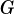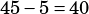5G + 45R = 380

At a school fair, students can win colored tokens that are worth a different number of points depending on the color. One student won G green tokens and R red tokens worth a total of 380 points. The given equation represents this situation. How many more points is a red token worth than a green token?

This is a “translating between words and math” question. Ifandrepresent the number of green and red tokens, respectively, and we know this particular students total tokens are worth the 380 on the right side of the equation, then the coefficients on the left hand side, the 5 and the 45, must be how much each individual green and red token are worth: a green token is worth 5 points, and a red token is worth 45 points.

The question asks us how many more points red is worth compared to green, so we subtract: